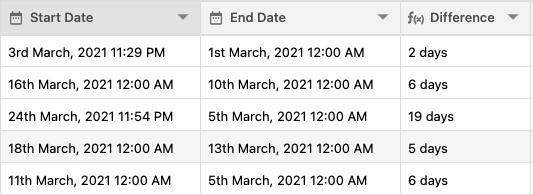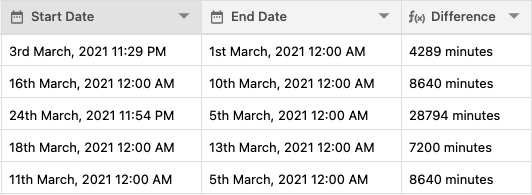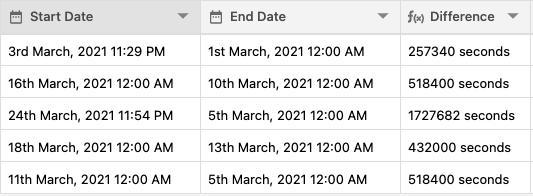You can find the difference between two dates using DATE_DIFF formula function in Stackby.

Learn about the complete formula guide here

DATE_DIFF({Start Date}, {End Date}, "days")DATE_DIFF({Start Date}, {End Date}, "minutes")DATE_DIFF({Start Date}, {End Date}, "seconds")